# Introduction

This is a continuation of the article Last time. This time I will write a script to move the created 3D object.

# Write an agent script

This time, we will control the pole by applying force to the cart of the cart pole. In other words, the cart becomes an agent to learn.
First of all, create a C # Script called CartPoleAgent in the Script folder.

In the agent script, inherit the Agent class and write the following functions.

–Initialize (): Get initial value
–CollectObservations (): Send observation data to sensor
–OnEpisodeBegin (): Determine the conditions for starting the step
–Heuristic (): Operate from the keyboard
–SetResetParameters (): Reset parameters

# Whole CartPoleAgent.cs

First, let’s put the entire code of the script.

``````
using UnityEngine;
using Unity.MLAgents;
using Unity.MLAgents.Sensors;
using System.Collections;
using System.Collections.Generic;

public class CartPoleAgent : Agent
{

//For learning
public GameObject pole;
Rigidbody poleRB;
Rigidbody cartRB;
EnvironmentParameters m_ResetParams;

//initial value
public override void Initialize()
{
//Initialization of learning
poleRB = pole.GetComponent<Rigidbody>();
cartRB = gameObject.GetComponent<Rigidbody>();
SetResetParameters();
}

//Send data to the sensor
public override void CollectObservations(VectorSensor sensor)
{
}
//Action at each step
{
//Apply force to the cart
var actionZ = 200f * Mathf.Clamp(verctorAction, -1f, 1f);

//Cart position, pole angle and angular velocity
float cart_z = this.gameObject.transform.localPosition.z;
float angle_x = pole.transform.localRotation.eulerAngles.x;

//angle_z-180~Convert to 180
if(180f < angle_x && angle_x < 360f)
{
angle_x = angle_x - 360f;
}

//The cart+-Reward if not 45 degrees+0.1 Other than that-1
if((-180f < angle_x && angle_x < -45f) || (45f < angle_x && angle_x < 180f))
{
SetReward(-1.0f);
EndEpisode();
}
else{
SetReward(0.1f);
}
//The position of the cart is-10~Reward if you exceed the range of 10-1
if(cart_z < -10f || 10f < cart_z)
{
SetReward(-1.0f);
EndEpisode();
}
}

//Determine initial conditions for step start
public override void OnEpisodeBegin()
{
//Reset agent state
gameObject.transform.localPosition = new Vector3(0f, 0f, 0f);
pole.transform.localPosition = new Vector3(0f, 2.5f, 0f);
pole.transform.localRotation = Quaternion.Euler(0f, 0f, 0f);
poleRB.angularVelocity = new Vector3(0f, 0f, 0f);
poleRB.velocity = new Vector3(0f, 0f, 0f);
//Give the pole a random tilt
poleRB.angularVelocity = new Vector3(Random.Range(-0.1f, 0.1f), 0f, 0f);
SetResetParameters();
}

//When operating from the keyboard
public override void Heuristic(float[] actionsOut)
{
actionsOut = Input.GetAxis("Horizontal");
}

//Reset pole conditions
public void SetPole()
{
poleRB.mass = m_ResetParams.GetWithDefault("mass", 1.0f);
pole.transform.localScale = new Vector3(0.4f, 2f, 0.4f);
}

//Function to reset parameters
public void SetResetParameters()
{
SetPole();
}
}
``````
``````public class CartPoleAgent : Agent
``````

Inherit the Agent class.

``````public GameObject pole;
Rigidbody poleRB;
Rigidbody cartRB;
EnviromentParameters m_ResetParams;
``````

Define a variable that contains the Pole object and the rigidbody of Cart and Pole. Since the Pole object is obtained from outside the script, make it public.

Initialize()
Initialize () gets the initial value of learning. This time it’s the Cart and Pole rigidbody and environment parameters. Also, the last line resets each parameter.

``````public override void Initialize()
{
//Initialization of learning
poleRB = pole.GetComponent<Rigidbody>();
cartRB = gameObject.GetComponent<Rigidbody>();
SetResetParameters();
}
``````

CollectObservations()
CollectionObservations () adds the observation information obtained by the agent to the sensor.

``````public override void CollectObservations(VectorSensor sensor)
{
}
``````

This time, from the top, “Cart position”, “Cart speed”, “Pole angle”, and “Pole angular velocity” are given.
The choice of this value depends on the training model. I’m not sure if this value is appropriate, so give it a try.

OnActionReceived () describes the behavior of the agent at each step. This time, we will apply force in the moving direction to the cart.
You can also set rewards here.

``````//Action at each step
{
//Apply force to the cart
var actionZ = 200f * Mathf.Clamp(verctorAction, -1f, 1f);

//Cart position, pole angle and angular velocity
float cart_z = this.gameObject.transform.localPosition.z;
float angle_x = pole.transform.localRotation.eulerAngles.x;

//angle_z-180~Convert to 180
if(180f < angle_x && angle_x < 360f)
{
angle_x = angle_x - 360f;
}

//The cart+-Reward if not 45 degrees+0.1 Other than that-1
if((-180f < angle_x && angle_x < -45f) || (45f < angle_x && angle_x < 180f))
{
SetReward(-1.0f);
EndEpisode();
}
else{
SetReward(0.1f);
}
//The position of the cart is-10~Reward if you exceed the range of 10-1
if(cart_z < -10f || 10f < cart_z)
{
SetReward(-1.0f);
EndEpisode();
}
}
``````

The code that moves the cart. The input value is vectorAction. Convert this value to -200 to 200 to make it the magnitude of the applied force. The range of this value is appropriate. You can add force to the cart by playing with the Add Force of the rigid body of the Cart. This time, apply force in the Z direction.

``````//Apply force to the cart
var actionZ = 200f * Mathf.Clamp(verctorAction, -1f, 1f);
``````

Code for agent (cart) rewards. This time, if the pole angle is within -45 ° to 45 °, the reward will be +0.1, otherwise the reward will be -1.0 and the game will end. Also, if the position of the cart goes out of the range of -10 to 10 so that the cart does not move too much sideways, a reward of -1.0 will be given and the game will end.

``````//The cart+-Reward if not 45 degrees+0.1 Other than that-1
if((-180f < angle_x && angle_x < -45f) || (45f < angle_x && angle_x < 180f))
{
SetReward(-1.0f);
EndEpisode();
}
else{
SetReward(0.1f);
}
//The position of the cart is-10~Reward if you exceed the range of 10-1
if(cart_z < -10f || 10f < cart_z)
{
SetReward(-1.0f);
EndEpisode();
}
``````

OnEpisodeBegin()
OnEpisodeBegin () determines the initial conditions of the game. This time the cart is returned to its initial position, giving the pole a random tilt.

``````//Determine initial conditions for step start
public override void OnEpisodeBegin()
{
//Reset agent state
gameObject.transform.localPosition = new Vector3(0f, 0f, 0f);
pole.transform.localPosition = new Vector3(0f, 2.5f, 0f);
pole.transform.localRotation = Quaternion.Euler(0f, 0f, 0f);
poleRB.angularVelocity = new Vector3(0f, 0f, 0f);
poleRB.velocity = new Vector3(0f, 0f, 0f);
//Give the pole a random tilt
poleRB.angularVelocity = new Vector3(Random.Range(-0.1f, 0.1f), 0f, 0f);
SetResetParameters();
}
``````

Heuristic()
Heuristic () is used to move the model by keyboard input. This time, it corresponds to the left and right input of the cross key.

``````//When operating from the keyboard
public override void Heuristic(float[] actionsOut)
{
actionsOut = Input.GetAxis("Horizontal");
}
``````

# Set Behavior Parameters, try to actually move

Also, add Behavior Parameters and Decision Requester from Add Component. This is required during reinforcement learning.

You can change the mode of the model with Behavior Parameters / Behavior Type. This time I want to use keyboard input, so set it to Heuristic Only. Set to Defaults when training and Inference Only when using a trained model. (If you want to use Inference Only, you need to add the trained model to Model.)
Set the parameters as shown in the image below.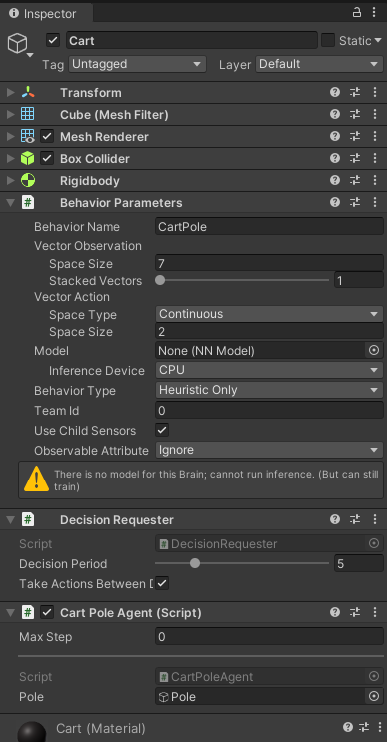If you run the model in this state, you can operate it with the keyboard. It is OK if it is firmly reset at the angle of the pole.

At this point, all the necessary preparations for learning have been completed. After that, if it is learned in the same way as the sample, it will be completed.

# To learn

Let’s actually learn the cart pole. Set Behavior Parameters / Behavior Type to Defaults for learning.
In addition, multiple cart poles will be used to improve learning efficiency. This time I made 10 units. You can easily increase it with copy and paste.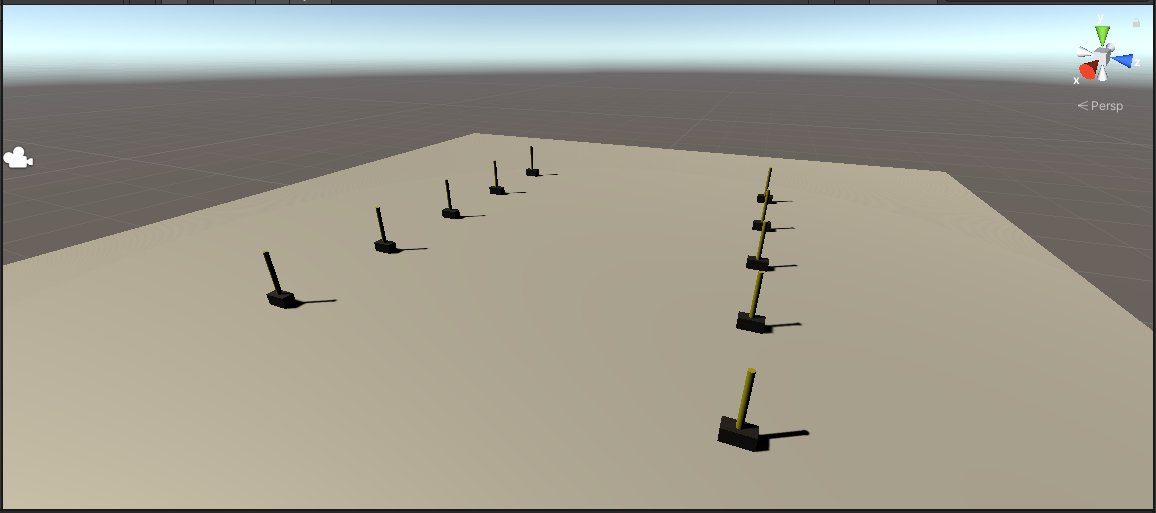Create a YAML file in the config folder to set the learning parameters. I referred to the parameters of 3D Ball.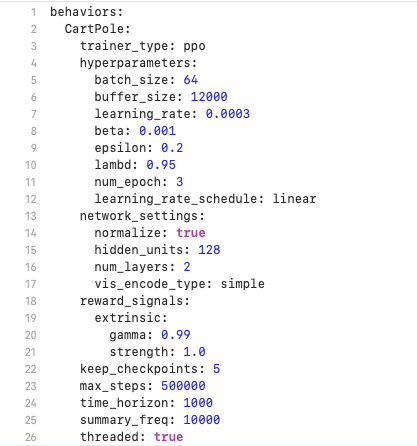Learning is completed in about 250,000 times. When the training is complete, a file called CartPole.nn will be created. Add this file to TFModels.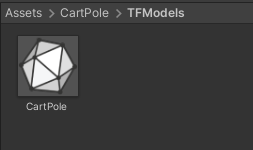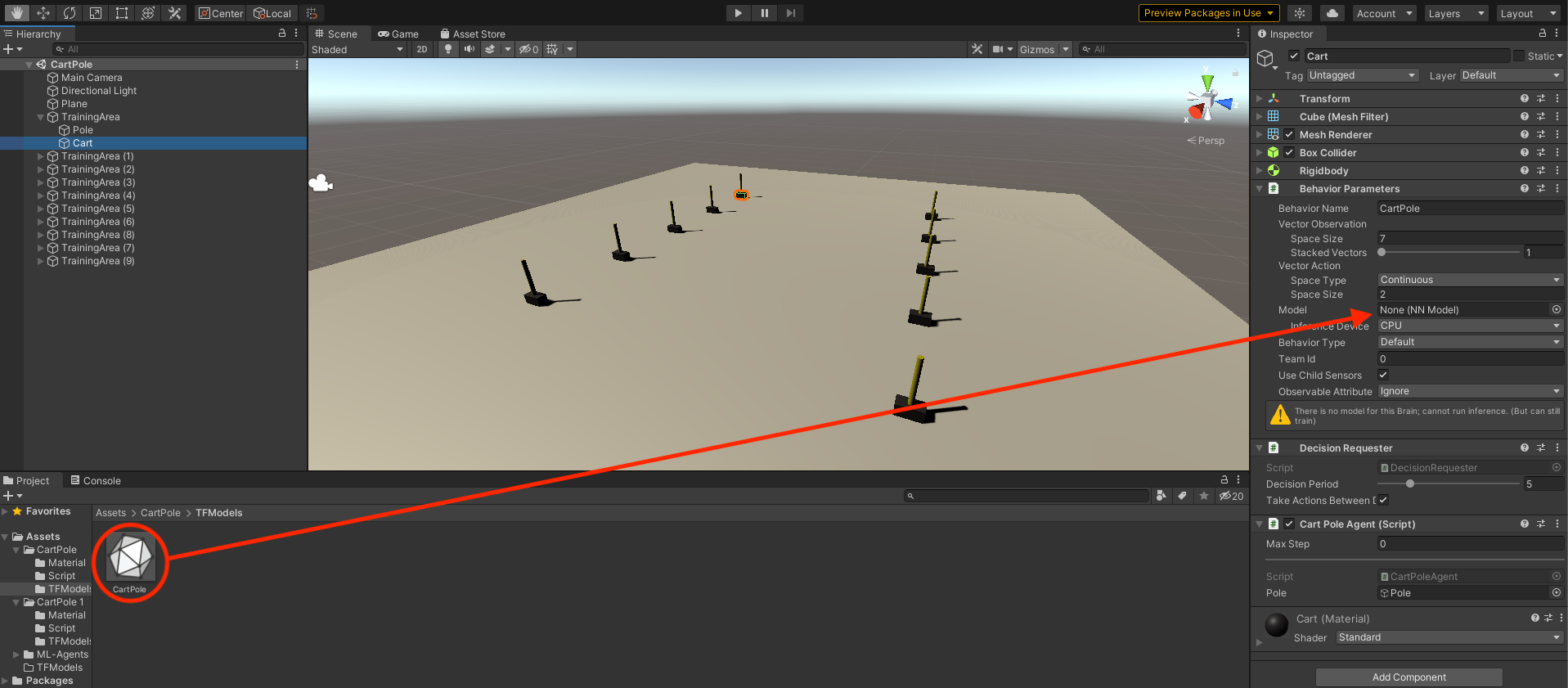This is a video of running a trained model with TrainingArea (1) to (9) inactive. You can see that you can control the pole so that it does not fall.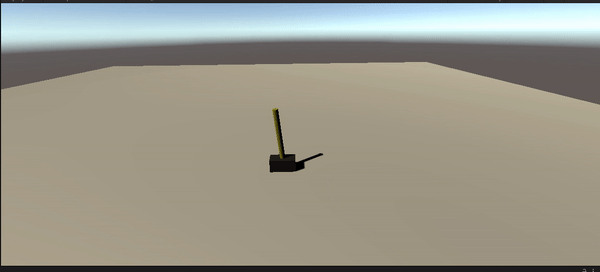# Summary

I made a model of Cartpole and trained it. With ML-Agents, you can easily implement a reinforcement learning model simply by writing an agent script. There are many things I don’t understand yet, so I would like to write an article again as my knowledge increases.

Tags:

Updated: